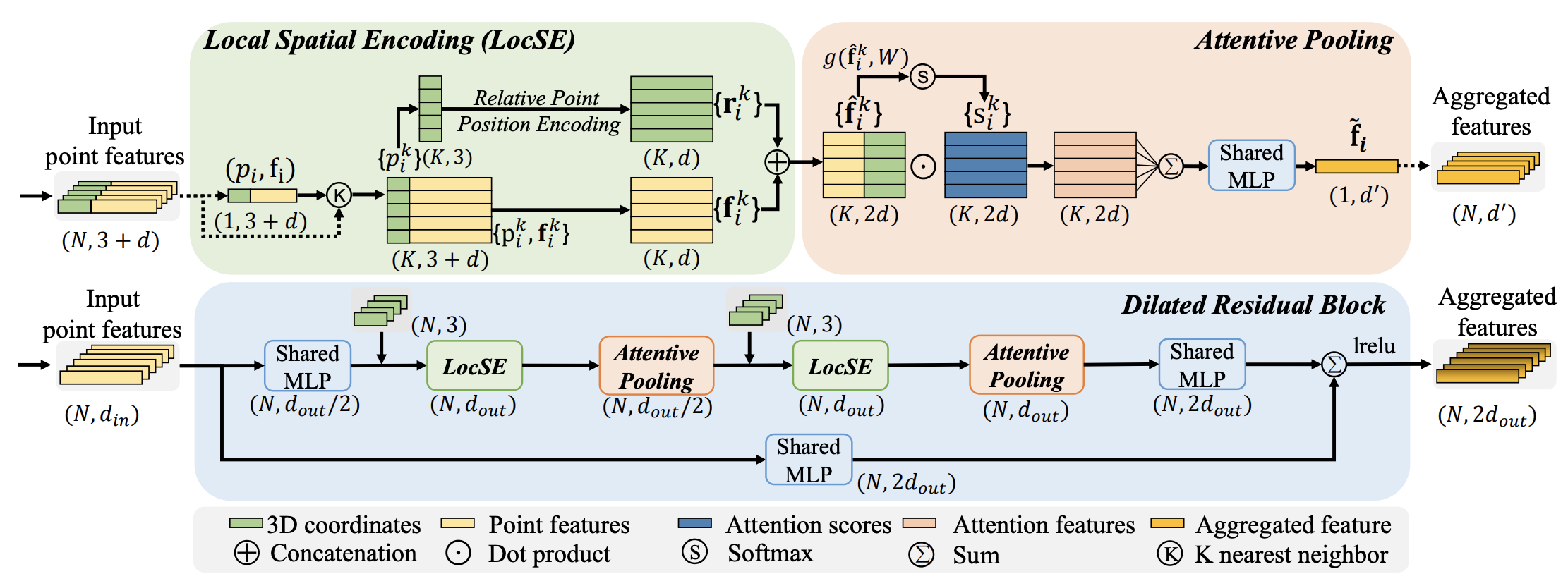# open3d.ml.tf.models.RandLANet¶

class open3d.ml.tf.models.RandLANet(*args, **kwargs)

Class defining RandLANet, a Semantic Segmentation model. Based on the architecture from the paper RandLA-Net: Efficient Semantic Segmentation of Large-Scale Point Clouds.

RandLA-Net is an efficient and lightweight neural architecture which directly infer per-point semantics for large-scale point clouds. The key approach is to use random point sampling instead of more complex point selection approaches. Although remarkably computation and memory efficient, random sampling can discard key features by chance. To overcome this, we introduce a novel local feature aggregation module to progressively increase the receptive field for each 3D point, thereby effectively preserving geometric details.

ArchitectureReferences

https://github.com/QingyongHu/RandLA-Net

__init__(name='RandLANet', num_neighbors=16, num_layers=4, num_points=45056, num_classes=19, ignored_label_inds=, sub_sampling_ratio=[4, 4, 4, 4], in_channels=3, dim_features=8, dim_output=[16, 64, 128, 256], grid_size=0.06, batcher='DefaultBatcher', ckpt_path=None, augment=None, **kwargs)

Initialize self. See help(type(self)) for accurate signature.

call(inputs, training=True)

Calls the model on new inputs.

In this case call just reapplies all ops in the graph to the new inputs (e.g. build a new computational graph from the provided inputs).

Note: This method should not be called directly. It is only meant to be overridden when subclassing tf.keras.Model. To call a model on an input, always use the __call__ method, i.e. model(inputs), which relies on the underlying call method.

Parameters
• inputs – A tensor or list of tensors.

• training – Boolean or boolean scalar tensor, indicating whether to run the Network in training mode or inference mode.

Returns

A tensor if there is a single output, or a list of tensors if there are more than one outputs.

static gather_neighbour(pc, neighbor_idx)
get_batch_gen(dataset, steps_per_epoch=None, batch_size=1)
get_loss(Loss, results, inputs)

Calculate the loss on output of the model.

Parameters
• Loss – Object of type SemSegLoss.

• results – Output of the model (B, N, C).

• inputs – Input of the model.

• device – device(cpu or cuda).

Returns

Returns loss, labels and scores.

get_optimizer(cfg_pipeline)

Returns an optimizer object for the model.

Parameters

cfg_pipeline – A Config object with the configuration of the pipeline.

Returns

Returns a new optimizer object.

inference_begin(data)

Function called right before running inference.

Parameters

data – A data from the dataset.

inference_end(results)

This function is called after the inference.

This function can be implemented to apply post-processing on the network outputs.

Parameters

results – The model outputs as returned by the call() function. Post-processing is applied on this object.

Returns

Returns True if the inference is complete and otherwise False. Returning False can be used to implement inference for large point clouds which require multiple passes.

inference_preprocess()

This function prepares the inputs for the model.

Returns

The inputs to be consumed by the call() function of the model.

static nearest_interpolation(feature, interp_idx)
Parameters
• feature – [B, d, N] input features matrix

• interp_idx – [B, up_num_points, 1] nearest neighbour index

Returns

[B, up_num_points, d] interpolated features matrix

preprocess(data, attr)

Data preprocessing function.

This function is called before training to preprocess the data from a dataset.

Parameters
• data – A sample from the dataset.

• attr – The corresponding attributes.

Returns

Returns the preprocessed data

static random_sample(feature, pool_idx)
Parameters
• feature – [B, d, N, 1] input features matrix

• pool_idx – [B, N’, max_num] N’ < N, N’ is the selected position after pooling

Returns

pool_features = [B, N’, d] pooled features matrix

transform(pc, feat, label)

Transform function for the point cloud and features.

Parameters

args – A list of tf Tensors.

transform_inference(data, min_possibility_idx)